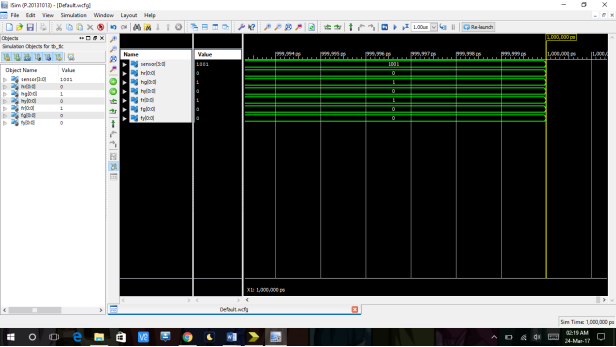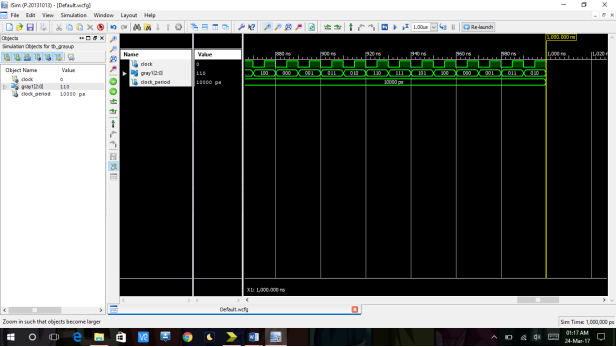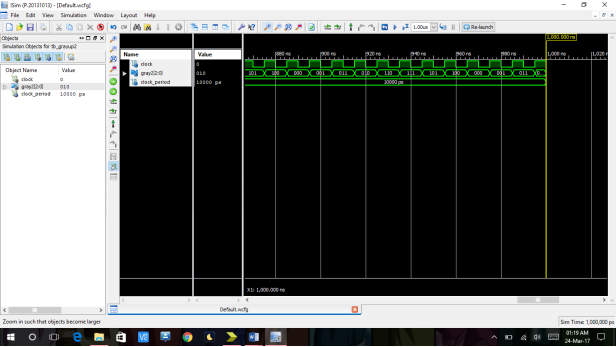# Traffic Light Controller System Design

1304 words (5 pages) Essay

31st Aug 2017 Engineering Reference this

Tags:

Disclaimer: This work has been submitted by a university student. This is not an example of the work produced by our Essay Writing Service. You can view samples of our professional work here.

Any opinions, findings, conclusions or recommendations expressed in this material are those of the authors and do not necessarily reflect the views of UKEssays.com.

library IEEE;

use IEEE.STD_LOGIC_1164.ALL;

use ieee.std_logic_unsigned.all;

— Uncomment the following library declaration if using

— arithmetic functions with Signed or Unsigned values

–use IEEE.NUMERIC_STD.ALL;

— Uncomment the following library declaration if instantiating

— any Xilinx primitives in this code.

–library UNISIM;

–use UNISIM.VComponents.all;

entity tlc is

Port ( sensor : in  STD_LOGIC_VECTOR (3 downto 0);

hr : out  STD_LOGIC_VECTOR (0 downto 0) ;

hg : out  STD_LOGIC_VECTOR (0 downto 0) ;

hy : out  STD_LOGIC_VECTOR (0 downto 0) ;

fr : out  STD_LOGIC_VECTOR (0 downto 0) ;

fg : out  STD_LOGIC_VECTOR (0 downto 0) ;

fy : out  STD_LOGIC_VECTOR (0 downto 0) );

end tlc;

architecture Behavioral of tlc is

signal ts : std_logic_vector(3 downto 0);

signal tm : std_logic_vector(3 downto 0);

signal tl : std_logic_vector(3 downto 0);

type state_type is (s0,s1,s2);

signal state : state_type;

begin

process (sensor)

begin

if sensor <= “0000” then

state <= s0;

elsif sensor <= “0100” then

state <= s1;

elsif sensor <= “0110” then

state <= s2;

end if;

case state is

when s0 =>

hr <= “0”;

hy <= “0”;

hg <= “1”;

fr <= “1”;

fy <= “0”;

fg <= “0”;

when s1 =>

hy <= “1”;

hg <= “0”;

ts <= “0000”;

if ts <= “0010” then

hy <= “0”;

hr <= “1”;

else ts <= ts+1;

end if;

fr <= “0”;

fy <= “1”;

ts <= “0000”;

if ts <= “0010” then

fy <= “0”;

fg <= “1”;

else ts <= ts+1;

end if;

tm <= “0000”;

if tm<= “0111” then

hr <= “0”;

hy <= “1”;

else tm<= tm+1;

end if;

ts <= “0000”;

if ts <= “0010” then

hy <= “0”;

hg <= “1”;

else ts <= ts+1;

end if;

tl<=”0000″;

if tl<=”1111″ then

if (sensor <= 0100)  then

state <= s1;

elsif sensor >= 0100 then

state <= s2;

end if;

else

tl<=tl+1;

end if;

when s2 =>

hy <= “1”;

hg <= “0”;

ts <= “0000”;

if ts <= “0010” then

hr <= “1”;

hy <= “0”;

else ts <= ts+1;

end if;

fr <= “0”;

fy <= “1”;

ts <= “0000”;

if ts <= “0010” then

fy <= “0”;

fg <= “1”;

else ts <= ts+1;

end if;

tm <= “0000”;

if tm<= “0111” then

hr <= “0”;

hy <= “1”;

else

tm<= tm+1;

end if;

ts <= “0000”;

if ts <= “0010” then

hy <= “0”;

hg <= “1”;

else ts <= ts+1;

end if;

tl<=”0000″;

if tl<=”1111″ then

if (sensor <= 0100 ) then

state <= s1;

elsif sensor >= 0100 then

state <= s2;

end if;

else

tl<=tl+1;

end if;

end case;

end process;

end Behavioral;Q.2) — Module Name:    gray_up – Behavioral using states(gray counter)

—————- ——————————————————

library IEEE;

use IEEE.STD_LOGIC_1164.ALL;

entity gray_up is

Port (     clock : in  STD_LOGIC;

gray1 : out  STD_LOGIC_VECTOR (2 downto 0));

end gray_up;

architecture Behavioral of gray_up is

type state_type is (s0,s1,s2,s3,s4,s5,s6,s7);

signal state : state_type;

begin

process(clock)

begin

case state is

when s0 =>

gray1 <= “000”;

if (rising_edge (clock)) then

state <= s1;

end if;

when s1 =>

gray1 <= “001”;

if (rising_edge (clock)) then

state <= s2;

end if;

when s2 =>

gray1 <= “011”;

if (rising_edge (clock))

then state <= s3;

end if;

when s3 =>

gray1 <= “010”;

if (rising_edge (clock))

then state <= s4;

end if;

when s4 =>

gray1 <= “110”;

if (rising_edge (clock)) then state <= s5;

end if;

when s5 =>

gray1 <= “111”;

if (rising_edge (clock)) then state <= s6;

end if;

when s6 =>

gray1 <= “101”;

if (rising_edge (clock))

then state <= s7;

end if;

when s7 =>

gray1 <= “100”;

if (rising_edge (clock))

then state <= s0;

end if;

end case;

end process;

end Behavioral;————————————————————————-

— Module Name:    gray_up2 – Behavioral ( using counter)

————————————————————————-

library IEEE;

use IEEE.STD_LOGIC_1164.ALL;

use IEEE.STD_LOGIC_UNSIGNED.all;

entity gray_up2 is

Port (     clock : in  STD_LOGIC;

gray2 : out  STD_LOGIC_VECTOR (2 downto 0));

end gray_up2;

architecture Behavioral of gray_up2 is

signal count : std_logic_vector (2 downto 0) := “000”;

begin

process(clock)

begin

if rising_edge(clock) then

if (count = 111) then count <= “000”;

else

count <= (count + “1”);

end if;

gray2(0) <= (count(0) xor count(1));

gray2(1) <= (count(1) xor count(2));

gray2(2) <= (count(2));

end if;

end process;

end Behavioral;Comparison:

The non states Gray code takes Cpu time to complete 7.58 secs

Memory usage 300k kilo bytes

But the state machine grey counter only takes 5secs sec cpu time

With 256k kilobytes memory usage.

Hence, the state machines is considered better over non state machine.

## Related Services

View all

### DMCA / Removal Request

If you are the original writer of this essay and no longer wish to have your work published on the UKDiss.com website then please:

Related Services